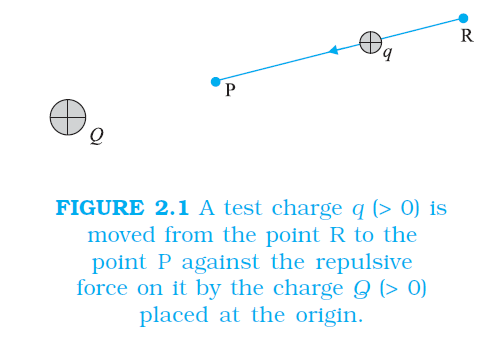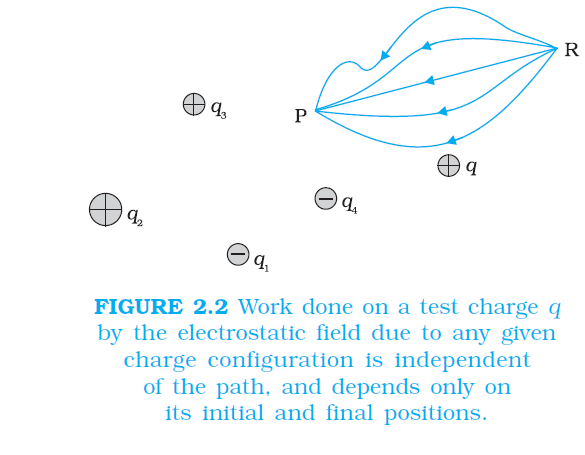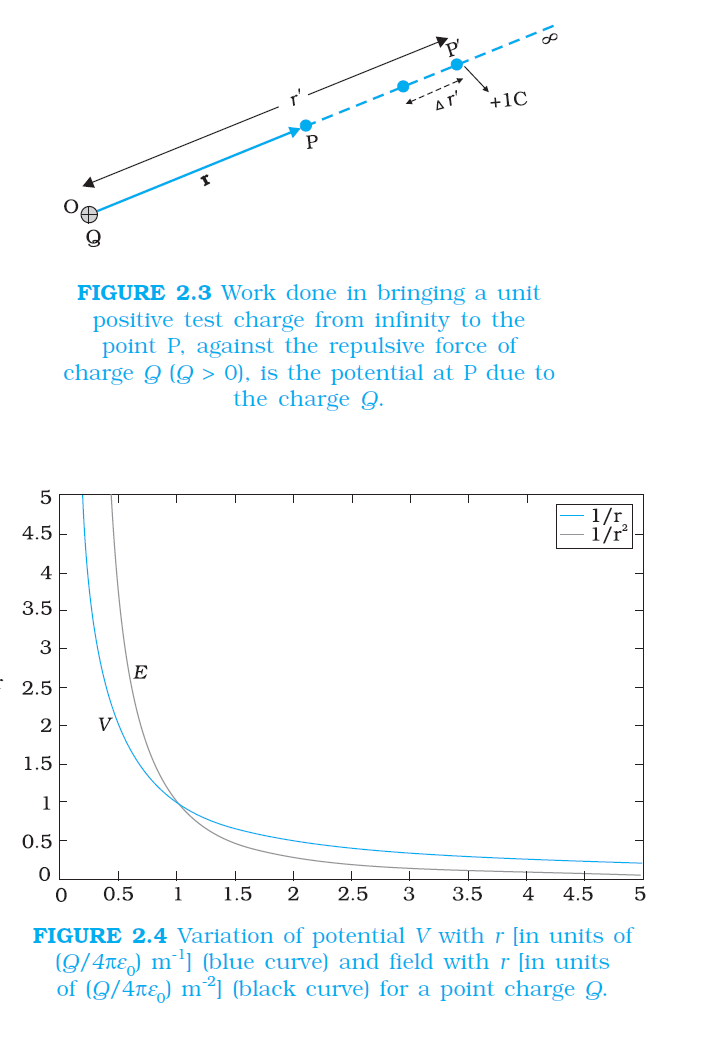Physics ELECTROSTATIC POTENTIAL
Click for Only Video

### Topic covered

color{blue}{star} INTRODUCTION
color{blue}{star} ELECTROSTATIC POTENTIAL
color{blue}{star} POTENTIAL DUE TO A POINT CHARGE

### Electrostatic Potential and Work done in an Electric Fieldcolor{blue} ✍️ When an external force does work in taking a body from a point to another against a force like spring force or gravitational force, that work gets stored as potential energy of the body.

color{blue} ✍️ When the external force is removed, the body moves, gaining kinetic energy and losing an equal amount of potential energy.

color{blue} ✍️ The sum of kinetic and potential energies is thus conserved. Forces of this kind are called "conservative forces." Spring force and gravitational force are examples of conservative forces.

color{blue} ✍️ Coulomb force between two (stationary) charges, like the gravitational force, is also a conservative force.

color{blue} ✍️ This is not surprising, since both have inverse-square dependence on distance and differ mainly in the proportionality constants – the masses in the gravitational law are replaced by charges in Coulomb’s law.

color{blue} ✍️Consider an electrostatic field E due to some charge configuration. First, for simplicity, consider the field E due to a charge Q placed at the origin.

color{blue} ✍️ Now, imagine that we bring a test charge q from a point R to a point P against the repulsive force on it due to the charge Q. With reference to Fig. 2.1, this will happen if Q and q are both positive or both negative. For definiteness, let us take Q, q > 0.color{blue} ✍️ First, we assume that the test charge q is so small that it does not disturb the original configuration, namely the charge Q at the origin (or else, we keep Q fixed at the origin by some unspecified force).

color{blue} ✍️ Second, in bringing the charge q from R to P, we apply an external force F_(ext) just enough to counter the repulsive electric force F_E (i.e, F_(ext)= –FE).

color{blue} ✍️ This means there is no net force on or acceleration of the charge q when it is brought from R to P, i.e., it is brought with infinitesimally slow constant speed. In this situation, work done by the external force is the negative of the work done by the electric force, and gets fully stored in the form of potential energy of the charge q.

color{blue} ✍️ If the external force is removed on reaching P, the electric force will take the charge away from Q – the stored energy (potential energy) at P is used to provide kinetic energy to the charge q in such a way that the sum of the kinetic and potential energies is conserved. Thus, work done by external forces in moving a charge q from R to P is

 W_(RP) = int_(R)^(P) F_(ext) * dr

color{blue}(= - int_(R)^(P) F_E * dr) .........2.1

color{blue} ✍️ This work done is against electrostatic repulsive force and gets stored as potential energy.

color{blue} ✍️ At every point in electric field, a particle with charge q possesses a certain electrostatic potential energy, this work done increases its potential energy by an amount equal to potential energy difference between points R and P.

color{blue} ✍️ Thus, potential energy difference

color{blue}(DeltaU=U_P -U_R = W_(RP)) ................2.2

(Note here that this displacement is in an opposite sense to the electric force and hence work done by electric field is negative, i.e., –W_(RP) .)

color{blue} ✍️ Therefore, we can define electric potential energy difference between two points as the work required to be done by an external force in moving (without accelerating) charge q from one point to another for electric field of any arbitrary charge configuration.

color{blue} ✍️ Two important comments may be made at this stage:

color{blue} {(i)} The right side of Eq. (2.2) depends only on the initial and final positions of the charge.

color{blue} ✍️ It means that the work done by an electrostatic field in moving a charge from one point to another depends only on the initial and the final points and is independent of the path taken to go from one point to the other.

color{blue} ✍️This is the fundamental characteristic of a conservative force.

color{blue} ✍️The concept of the potential energy would not be meaningful if the work depended on the path.

color{blue} ✍️The path-independence of work done by an electrostatic field can be proved using the Coulomb’s law. We omit this proof here.

color{blue} {(ii)} Equation (2.2) defines potential energy difference in terms of the physically meaningful quantity work. Clearly, potential energy so defined is undetermined to within an additive constant.

color{blue} ✍️ What this means is that the actual value of potential energy is not physically significant; it is only the difference of potential energy that is significant. We can always add an arbitrary constant α to potential energy at every point, since this will not change the potential energy difference:

color{blue}((U _P =alpha) - (U_(R) + alpha) = U_P - U_R)

color{blue} ✍️ Put it differently, there is a freedom in choosing the point where potential energy is zero. A convenient choice is to have electrostatic potential energy zero at infinity. With this choice, if we take the point R at infinity, we get from Eq. (2.2)

color{blue}(W_(∞P) = U_P - U∞ = U_P) ................2.3

color{blue} ✍️ Since the point P is arbitrary, Eq. (2.3) provides us with a definition of potential energy of a charge q at any point. Potential energy of charge q at a point (in the presence of field due to any charge configuration) is the work done by the external force (equal and opposite to the electric force) in bringing the charge q from infinity to that point.

### ELECTROSTATIC POTENTIALcolor{blue} ✍️ Consider any general static charge configuration. We define potential energy of a test charge q in terms of the work done on the charge q.

color{blue} ✍️ This work is obviously proportional to q, since the force at any point is qE, where E is the electric field at that point due to the given charge configuration.

color{blue} ✍️ It is, therefore, convenient to divide the work by the amount of charge q, so that the resulting quantity is independent of q,In other words, "work done per unit test charge is characteristic of the electric field associated with the charge configuration."

color{blue} ✍️ This leads to the idea of electrostatic potential V due to a given charge configuration. From Eq. (2.1), we get: Work done by external force in bringing a unit positive charge from point R to P

color{blue}(V_P - V_R (= (U_P-U_R)/q))

......................... 2.4

color{blue} ✍️where V_P and V_R are the electrostatic potentials at P and R, respectively. Note, as before, that it is not the actual value of potential but the potential difference that is physically significant. If, as before, we choose the potential to be zero at infinity, Eq. (2.4) implies:

color{blue} ✍️ Work done by an external force in bringing a unit positive charge from infinity to a point = electrostatic potential (V ) at that point.

color{blue} ✍️ Note, as before, that it is not the actual value of potential but the potential difference that is physically significant. If, as before, we choose the potential to be zero at infinity, Eq. (2.4) implies:

color{blue} ✍️ Work done by an external force in bringing a unit positive charge from infinity to a point = electrostatic potential (V ) at that point.

color{blue} ✍️ In other words, the electrostatic potential (V ) at any point in a region with electrostatic field is the work done in bringing a unit positive charge (without acceleration) from infinity to that point.

color{blue} ✍️ The qualifying remarks made earlier regarding potential energy also apply to the definition of potential. To obtain the work done per unit test charge, we should take an infinitesimal test charge δq, obtain the work done δW in bringing it from infinity to the point and determine the ratio (δW)/(δq).

color{blue} ✍️ Also, the external force at every point of the path is to be equal and opposite to the electrostatic force on the test charge at that point.### POTENTIAL DUE TO A POINT CHARGEcolor{blue} ✍️ Consider a point charge Q at the origin (Fig. 2.3). For definiteness, take Q to be positive. We wish to determine the potential at any point P with position vector r from the origin.

color{blue} ✍️ For that we must calculate the work done in bringing a unit positive test charge from infinity to the point P.

color{blue} ✍️ For Q > 0, the work done against the repulsive force on the test charge is positive. Since work done is independent of the path, we choose a convenient path – along the radial direction from infinity to the point P.color{blue} ✍️ At some intermediate point P′ on the path, the electrostatic force on a unit positive charge is

color{blue}((Qxx1)/(4piepsilon_0r'^2) hatr') ..........2.5

=> where hatr′ is the unit vector along OP′. Work done against this force from r′ to r′ + Δr′ is

color{blue}(DeltaW = - (Q)/(4piepsilon_0r^'a) hatr') ................2.6

color{blue} ✍️ The negative sign appears because for color{blue}(Δr ′ < 0, ΔW) is positive . Total work done (W) by the external force is obtained by integrating Eq. (2.6) from color{blue}(r′ = ∞ to r′ = r,)

color{blue}(W = - int_(∞)^(r) (Q)/(4piepsilon_0(r')^2) dr' = (Q)/(4piepsilon_0r') |_(∞)^(r) = (Q)/(4piepsilon_0r)) ..........2.7

color{blue} ✍️This, by definition is the potential at P due to the charge Q

color{blue}(V(r) = (Q)/(4piepsilon_0r))

................ 2.8

color{blue} ✍️ Equation (2.8) is true for any sign of the charge Q, though we considered Q > 0 in its derivation. For Q < 0, V < 0, i.e., work done (by the external force) per unit positive test charge in bringing it from infinity to the point is negative.

color{blue} ✍️ This is equivalent to saying that work done by the electrostatic force in bringing the unit positive charge form infinity to the point P is positive.

color{blue} ✍️ This is as it should be, since for Q < 0, the force on a unit positive test charge is attractive, so that the electrostatic force and the displacement (from infinity to P) are in the same direction. Finally, we note that Eq. (2.8) is consistent with the choice that potential at infinity be zero.

color{blue} ✍️ Figure (2.4) shows how the electrostatic potential color{blue}((∝1//r)) and the electrostatic field color{blue}(∝ 1//r 2 )) varies with r.Q 3184580457(a) Calculate the potential at a point P due to a charge of 4 × 10^(–7) C located 9 cm away.

(b) Hence obtain the work done in bringing a charge of 2 × 10–9 C from infinity to the point P. Does the answer depend on the path along which the charge is brought?
Class 12 Chapter example 1Solution:

(a) color{blue}{V = 1/(4piepsilon_0) Q/r} = 9xx10^9 Nm ^2 C^(-2) xx(4xx10^(-7)C)/(0.09m)

= 4 xx 10^4 V

(b) color{blue}{W = qV} = 2 xx10^(−9) C xx 4 xx 10^4 V

= 8 × 10^(–5) J
No, work done will be path independent. Any arbitrary infinitesimal path can be resolved into two perpendicular displacements: One along r and another perpendicular to r. The work done corresponding to the later will be zero.